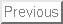# Computer Science 547 - Discrete Mathematics Prof. Steven Skiena Spring 1999

Homework 4
Due Thursday, March 25, 1999

Each of the problems should be solved on a separate sheet of paper to facilitate grading. Limit the solution of each problem to one sheet of paper. Staple all these sheets together! Please don't wait until the last minute to look at the problems.

Do the following exercises in Graham, Knuth, and Patashnik:

1.
Exercise 7-7.

2.
Exercise 7-21(a).
3.

Exercise 7-23.

4.
Warmup exercises 8:1-6.

5.
Exercise 8-7.

6.
Exercise 8-11.

7.
Exercise 8-15.

8.
Let,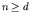be a constant coefficient recurrence relation of history d, with specified initial conditions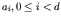.

(a) Let fn = c0, for some constant c0. Does there necessarily exist a constant coefficient homogeneous recurrence relation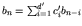where an = bn for all n. If so, prove it, and give d' in terms of d. If not, give a counter-example.

(b) Let fn = c0n, for some constant c0. Does there necessarily exist a constant coefficient homogeneous recurrence relation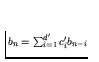where an = bn for all n. If so, prove it, and give d' in terms of d. If not, give a counter-example.

(c) Let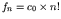, for some constant c0. Does there necessarily exist a constant coefficient homogeneous recurrence relationwhere an = bn for all n. If so, prove it, and give d' in terms of d. If not, give a counter-example.

9.
Use generating functions to solve the recurrence:

An = 3 An-1 - 2 An-2 + 3

where A0 = A1 = 1.

10.
Use generating functions to solve the recurrence:

where A0 = 2 and A1 = -1.